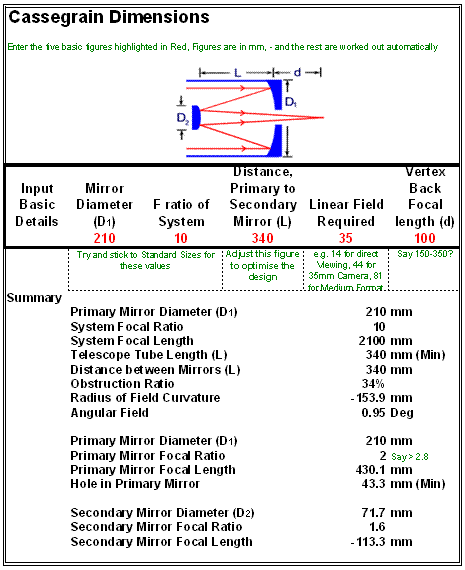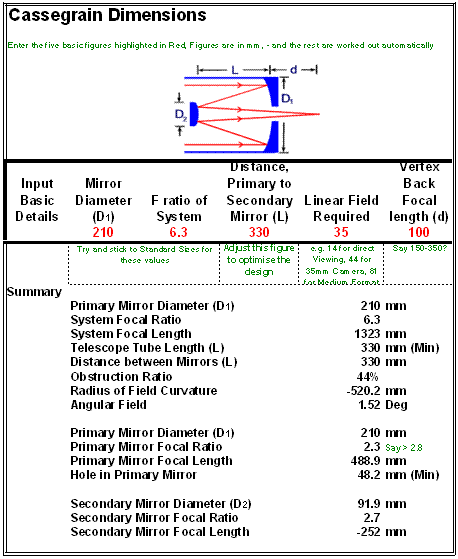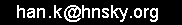# Classic 8 inch LX200 optical parameters and curvature

Meade does not provide much information about the classic LX200 Schmidt Casssegrain telescope. This page tries to establish them by reverse engineering.

What's available from Meade is the following:The primary mirror is F/1,9 so for an 8,25inch diameter the focal length is 8.25*1.8 equals 15.675 inch or 398 mm.

For estimating the Petzval field curvature, it is safe to ignore the Schmidt plate and assume the telescope as a simple cassegrain type. Measuring my own native F/6,3, I estimate the distance between the corrector plate and mirror about 330 to 340 mm.

The dimensions can be worked out on squared paper, Oldham's spreadsheet or demo optical design software from Atmos or Modas:

For the 8 inch, F/10 Oldams's spreadsheet comes with the following solution:Same down for the F/6,3:The math to calculate curvature  is described at   http://www.telescope-optics.net/curvature.htm  The Petzval curvature for a pair of mirrors is given by formula (30) where the 1/radiusPetzval equals   2/radiusmirror2 - 2/radiusmirror1. The factor 2 is introduced while the mirror radius is two times lager then the mirror focus. The formula is based on the Petzval’s theorem. The 1/curvature of the Petzval surface in a system is the sum of the 1/powers of these surfaces. For mirrors this the sum of  1/focal length of each mirror. so (30) can be rewritten as 1/radius Petzval equals   1/focallengthmirror2 - 1/focallengthmirror1.

Putting the data in a spreadsheet calculation gives the same results for the curvature.

So resume,  the estimated field curvature of a 8 inch LX200 F/10 is about -150 mm and a 8 inch LX200 native F/6,3 is about  -500 mm. The field curvature of the native F/6,3 is about a factor 3 better then the F/10!

A double check of the data in the optical design program Modas gave simular results:
 Telescope Observed object height from telescope centre Projection height on CCD from centre Focus error from focussed centre 8"  LX200 native F/6,3 0.5 degrees 10.9 mm 0.094 mm 8"  LX200 F/10 0.31 degrees 10.8 mm 0.28 mm

The curvature of a Schmidt Cassegrain is similar as a refractor concave, curved towards the primary mirror.

The curvature of a refractor is about 1/3 of the focal length. So maybe resulting in a curvature of 150, 200 or 250 mm. Looks like a  refractor field flattener could help for a F/10 Schmidt Cassegrain with a curvature of 150 mm but not really for a native F/6,3 with a curvature of 500 mm. Then there is still the problem of coma.

Commercial 6" and 8" Ritchey-Chrétien telescopes with a focal ratio of F/9 or F/8 should to have a curvature somewhere halfway between the F/10 and F/6,3 Schmidt Cassegrain so maybe somewhere around 300 mm, so still near the range of refractor field flattener. ref 1)

Any comments, please send an email :